# NCERT Solutions for Class 8 Maths Chapter 13 Direct and Inverse Proportions Ex 13.2

These NCERT Solutions for Class 8 Maths Chapter 13 Direct and Inverse Proportions Ex 13.2 Questions and Answers are prepared by our highly skilled subject experts.

## NCERT Solutions for Class 8 Maths Chapter 13 Direct and Inverse Proportions Exercise 13.2

Question 1.
Which of the following are in inverse proportion?
(i) The number of workers on a job and the time to complete the job.
(ii) The time taken for a journey and the distance travelled in a uniform speed.
(iii) Area of cultivated land and the crop harvested.
(iv) The time taken for a fixed journey and the speed of the vehicle.
(v) The population of a country and the area of land per person.
(i) If the number of workers increases then time to complete the job would decrease.
∴ It is an inverse proportion.
(ii) The time taken for a journey and the distance travelled in a uniform speed are not an inverse proportion.
(iii) More the area of land, more the crops that would be harvested.
∴ It is not an inverse proportion.
(iv) If speed is more, time to cover a fixed distance would be less.
∴ It is an inverse proportion.
(v) For more population, less area per person would be required.
∴ It is an inverse proportion.Question 2.
In a Television game show, the prize money of ₹ 1,00,000 is to be divided equally amongst the winners. Complete the following table and find whether the prize money given to an individual winner is directly or inversely proportional to the number of winners?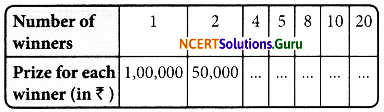More the number of winners, less would be the prize money.
∴ It is an inverse variation.
1 x 1,00,000 = 4 x x1
x1 = $$\frac{1 \times 1,00,000}{4}$$ = ₹ 25000
1 x 1,00,000 = 5 x x2
x2 = $$\frac{1 \times 1,00,000}{5}$$ = ₹ 20000
1 x 1,00,000 = 8 x x3
x3 = $$\frac{1 \times 1,00,000}{8}$$ = ₹ 12500
1 x 1,00,000 = 10 x x4
x4 = $$\frac{1 \times 1,00,000}{10}$$ = ₹ 10000
1 x 1,00,000 = 20 x x5
x5 = $$\frac{1 \times 1,00,000}{20}$$ = ₹ 5000
Thus, the table is completed as follows: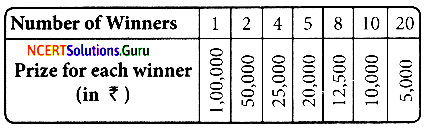Since 1 x 1,00,000 = 2 x 50,000 = 4 x 25,000 = 5 x 20,000
= 8 x 12500 = 10 x 10,000 = 20 x 5,000
So, the prize money given to an individual winner is inversely proportion to the number of winners.

Question 3.
Rehman is making a wheel using spokes. He wants to fix equal spokes in such a way that the angles between any pair of consecutive spokes are equal. Help him by completing the following table.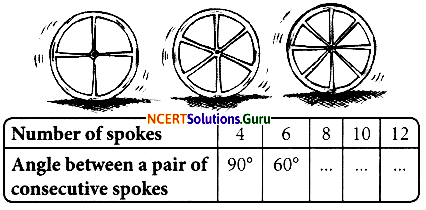(i) Are the number of spokes and the angles formed between the pairs of consecutive spokes in inverse proportion?
(ii) Calculate the angle between a pair of consecutive spokes on a wheel with 15 spokes.
(iii) How many spokes would be needed, if the angle between a pair of consecutive spokes is 40°?
(i) Obviously, more the number of spokes, less the measure of angle between a pair of consecutive spokes.
∴ It is an inverse variation
4 x 90 = 8 x x1
x1 = $$\frac{4 \times 90}{8}$$ =45°
4 x 90 = 10 x x2
x2 = $$\frac{4 \times 90}{10}$$ = 36°
4 x 90 = 12 x x3
x3 = $$\frac{4 \times 90}{12}$$ = 30°(ii) Let the required measure of angle be x°
∴ 15 x x° = 4 x 90°
∴ x = $$\frac{4 \times 90}{15}$$ = 24°
(iii) Let the required number of spokes be ‘n’
∴ n x 40° = 4 x 90°
n = $$\frac{4 \times 90^{\circ}}{40^{\circ}}$$
∴ The required number of spokes = 9.Question 4.
If a box of sweets is divided among 24 children, they will get 5 sweets each. How many would each get, if the number of the children is reduced by 4?
Let the number of sweets for each student be ‘x’Less the no. of students more the no. of sweets that each student will get.
∴ It is an inverse variation.
24 x 5 = 20 x x
x = $$\frac{24 \times 5}{20}$$ = 6
Hence, each student will get 6 sweets.

Question 5.
A farmer has enough food to feed 20 animals in his cattle for 6 days. How long would the food last if there were 10 more animals in his cattle?
Let the number of days the food last be YAs the number of animals increases, the number of days decreases.
∴ 20 x 6 = 30 x x
x = $$\frac{20 \times 6}{30}$$ = 4
Hence, the food would last for 4 days.

Question 6.
A contractor estimates that 3 persons could rewire Jasminder’s house in 4 days. If, he uses 4 persons instead of three, how long should they take to complete the job?
Let the required number of days be ‘x’If the number of persons increases, number of days decreases.
∴ It is an inverse proportion
3 x 4 = 4 x x 3×4
x = $$\frac{3 \times 4}{4}$$ = 3
∴ Hence, they would take 3 days to complete the job.Question 7.
A batch of bottles were packed in 25 boxes with 12 bottles in each box. If the same batch is packed using 20 bottles in each box, how many boxes would be filled?Let the required number of boxes be ‘x’.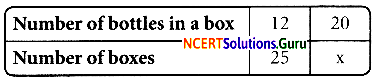More the number of bottles in a box, lesser will be the number of boxes that are required to be filled.
∴ It is an inverse variation.
12 x 25 = 20 x x
x = $$\frac{12 \times 25}{20}$$ = 15
∴ The required number of boxes = 15.

Question 8.
A factory requires 42 machines to produce a given number of articles in 63 days. How many machines would be required to produce the same number of articles in 54 days?
Let the required number of machines be ‘x’As number of machines increases, no. of days decreases.
∴ It is an inverse proportion
42 x 63 = x x 54
x = $$\frac{42 \times 63}{54}$$ = 49
∴ The required number of machines = 49.

Question 9.
A car takes 2 hours to reach a destination by travelling at the speed of 60 km/h. How long will it take when the car travels at the speed of 80 km/h?
Let the required number of hours be “x”.If the speed increases, the number of
hours decreases.
It is an inverse proportion
∴ 60 x 2 = 80 x x
x = $$\frac{60 \times 2}{80}$$ = — = 1$$\frac { 1 }{ 2 }$$
The time taken is 1$$\frac { 1 }{ 2 }$$ hours.Question 10.
Two persons could fit new windows in a house in 3 days.
(i) One of the persons fell ill before the work started. How long would the job take now?
(ii) How many persons would be needed to fit the windows in one day?
(i) Let the the required no. of days be x.As the number of persons decreases, number of days increases.
∴ It is an inverse proportion 2 x 3 = 1 x x
x = 2 x 3 = 6 days
One person will complete the work in 6 days.

(ii) Let the required number of persons be ‘x’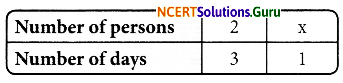As the number of days decreases, number of person increases.
It is a inverse proportion
2 x 3 = x x 1
x = $$\frac{2 \times 3}{1}$$ = 6
The required number of persons = 6.

Question 11.
A school has 8 periods a day each of 45 minutes duration. How long would each period be, if the school has 9 periods a day, assuming the number of school hours to be the same?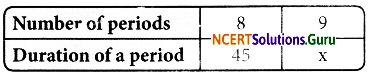x = $$\frac{8 \times 45}{9}$$ = 8 x 5 = 40 minutes